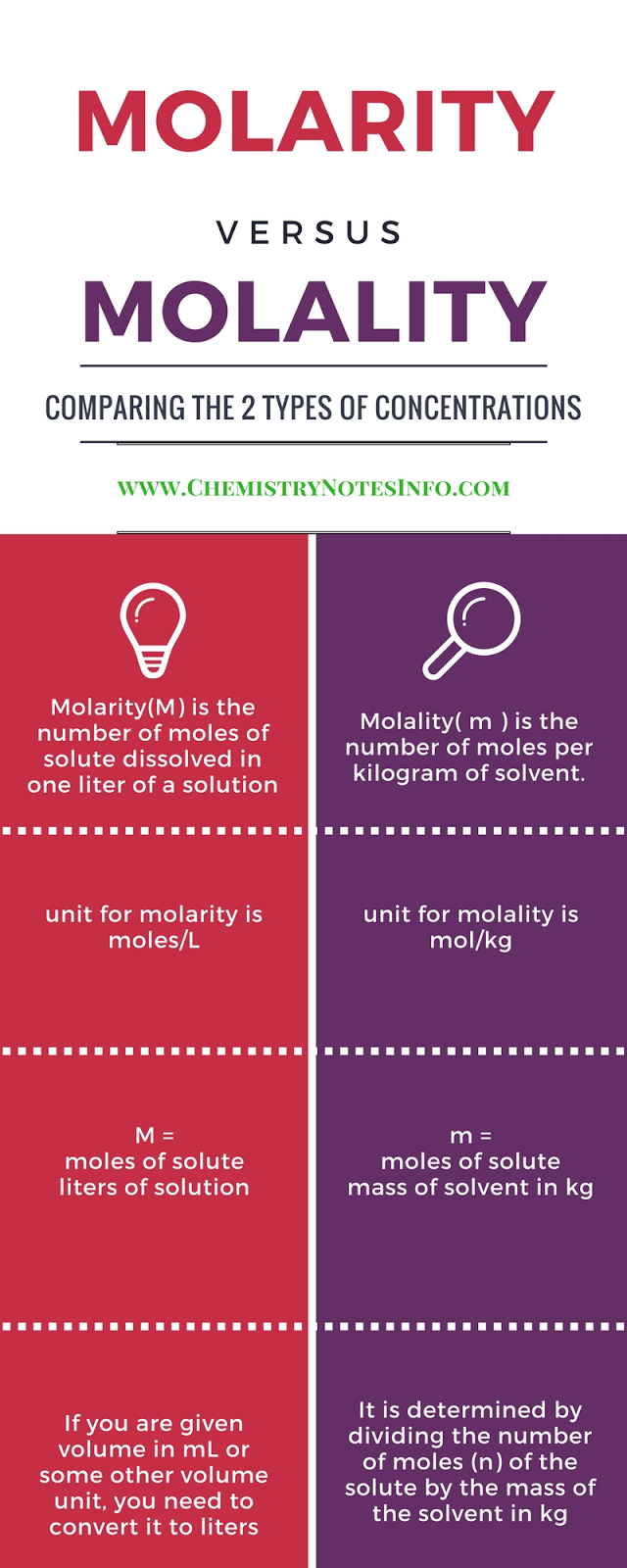# Wet-Chemistry

## What is Wet Chemistry ?

Wet Chemistry  is a branch of chemistry which deals with the study of and testing of chemicals in liquid state. Wetchemistry is also known as Bench Chemistry as many wet chemical methods are performed on chemistry laboratory benches.

# What is Normality ?

Normality is the ratio of gram equivalent weight to volume of solution in liter
where gram equivalent is the measure of reactive capacity of molecule consider for normality.

## Normality (N) :-

Gram equivalent weight  / Volume of the solution in liter.

Unit =>   N = "eq/L" (equivalent per liter)

## Aufbau Principle

According to this principle in the ground state of the atoms the orbital’s are filled in order of their increasing energies means electrons enter higher energy orbital’s  so order in which orbital’s are filled is 1s, 2s, 2p, 3s, 3p, 4s, 3d, 4p, 5s, 4d, 5p, 6s, 4f, 5d, 6p, 7s, 5f, 6d, 7p.

## Pauli Exclusion Principle

·         given by W. Pauli (1926).
·        Two electrons in an atoms can’t have same set of 4-quantum no.
·        Only two electrons may exist in same orbital and these electrons must have opposite spin.

## Hund’s Rule of Maximum Multiplicity

·        According to this rule pairing of  electron in the orbital’s belonging to the same sub-shell (p, d or f)
·        Does not take place until each orbital belonging to that sub-shell has got one electron each i.e. it is singly occupied.

# What is Molality?

It is the ratio of moles of substance to kilogram of solvent.
Where mole is weight in gram divided by molecular weight.
Molality is chemistry terminology.

## Molality (m) :-

No. of moles of the solute  Mass of solvent in Kilogram (Kg).

Unit =>   m = Moles/KgWhat is Molality

## NOTE:

If error occur=> Change website url from www.chemistrynotesinfo.blogspot.in to www.chemistrynotesinfo.com